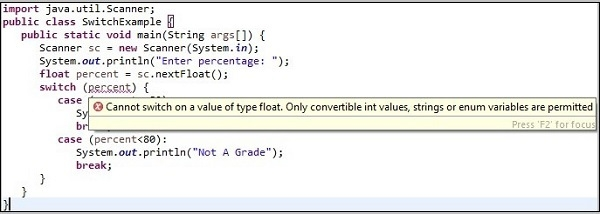# What are the rules to be followed while working with a switch statement in java?

A switch statement allows a variable to be tested for equality against a list of values. Each value is called a case, and the variable being switched on is checked for each case.

## Syntax

switch(expression) {
case value :
// Statements
break;
case value :
// Statements
break;
// You can have any number of case statements.
default :
// Statements
}

## Rules to be followed

While working with a switch statement keep the following points in mind −

• We must only use int, char or, enum types along with switch. Usage of any other types generates a compile time error.

## Example

import java.util.Scanner;
public class SwitchExample {
public static void main(String args[]) {
Scanner sc = new Scanner(System.in);
System.out.println("Enter percentage: ");
float percent = sc.nextFloat();
switch (percent) {
case (percent>=80):
break;
case (percent<80):
break;
}
}
}

## Compile time error

SwitchExample.java:7: error: incompatible types: possible lossy conversion from float to int
switch (percent) {
^
SwitchExample.java:8: error: constant expression required
case (percent>=80):
^
SwitchExample.java:11: error: constant expression required
case (percent<80):
^
3 errors

If you compile the above program in eclipse it shows a message as shown below −All the statements in a switch case are executed until they reach a break statement. Therefore, you need to have break after every case else all the cases will be executed irrespective of the option you choose.

## Example

Live Demo

import java.util.Scanner;
public class SwitchExample {
public static void main(String args[]) {
Scanner sc = new Scanner(System.in);
System.out.println("Available models: Activa125(act125), Activa5G(act5g)," + " Accesses125(acc125), Vespa(ves), TvsJupiter(jup)");
System.out.println("Select one model: ");
String model = sc.next();
switch (model) {
case "act125":
System.out.println("The price of activa125 is 80000");
//break;
case "act5g":
System.out.println("The price of activa5G is 75000");
//break;
case "acc125":
System.out.println("The price of access125 is 70000");
//break;
case "ves125":
System.out.println("The price of vespa is 90000");
//break;
case "jup":
System.out.println("The price of tvsjupiter is 73000");
//break;
default:
break;
}
}
}

## Output

Available models: Activa125(act125), Activa5G(act5g), Accesses125(acc125), Vespa(ves), TvsJupiter(jup)
Select one model:
act125
The price of activa125 is 80000
The price of activa5G is 75000
The price of access125 is 70000
The price of vespa is 90000
The price of tvsjupiter is 73000
Model not found

Expressions we use in the switch statement must be constant, if we use other expressions a compile time error will be generated.

## Example

import java.util.Scanner;
public class SwitchExample {
public static void main(String args[]) {
Scanner sc = new Scanner(System.in);
String str[] = {"act125", "act5g", "acc125"};
System.out.println("Available models: Activa125(act125), Activa5G(act5g), Accesses125(acc125)");
System.out.println("Select one model: ");
String model = sc.next();
switch (model) {
case str:
System.out.println("The price of activa125 is 80000");
break;
case str:
System.out.println("The price of activa5G is 75000");
break;
case str:
System.out.println("The price of access125 is 70000");
break;
}
}
}

## Output

SwitchExample.java:10: error: constant string expression required
case str:
^
SwitchExample.java:13: error: constant string expression required
case str:
^
SwitchExample.java:16: error: constant string expression required
case str:
^
3 errors

Two cases Must not have same value. If so, a compile time error will be generated.

## Example

import java.util.Scanner;
public class SwitchExample {
public static void main(String args[]) {
Scanner sc = new Scanner(System.in);
String str[] = {"act125", "act5g", "acc125"};
System.out.println("Available models: Activa125(act125), Activa5G(act5g), Accesses125(acc125)");
System.out.println("Select one model: ");
String model = sc.next();
switch (model) {
case "act125":
System.out.println("The price of activa125 is 80000");
break;
case "act125":
System.out.println("The price of activa5G is 75000");
break;
case "acc125":
System.out.println("The price of access125 is 70000");
break;
}
}
}

## Compile time error

SwitchExample.java:13: error: duplicate case label
case "act125":
^
1 error

You can have the default statement anywhere and, statements above cases never gets executed.